## Example Questions

### Example Question #2 : Percentage

Robert has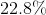of his cereal left.  Choose the answer which best expresses as a decimal, how much of his cereal he has eaten.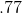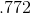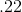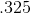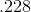Explanation:

The easiest way to convert between percent and decimal is to first convert the percentage into a fraction: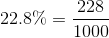Then, from the fraction, you can convert quite easily to a decimal:HOWEVER--in this problem, they are asking you for the portion that makes up the rest of the cereal--so you need to subtract your decimal above from, which yields the correct answer:### Example Question #10 : Percentage

Choose the answer below which best expresses the following as a decimal: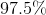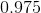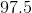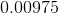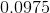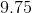Explanation:

When converting percents into decimals, know that the tens and ones digit of the percent will occupy the tenths and hundredths places of the decimal.  Any decimal following the whole percent will follow in sequence (i.e. the first decimal place in a percent corresponds to the thousandth place, and so on).  Therefore, ninety-seven point five percent is equal to: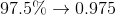### Example Question #11 : Percentage

Choose the answer which best expresses the following percentage as a decimal: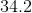%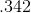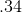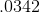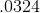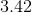To convert a percentage into a decimal, simply place the decimal point in front of the tens digit of the percentage.  So,% becomes.